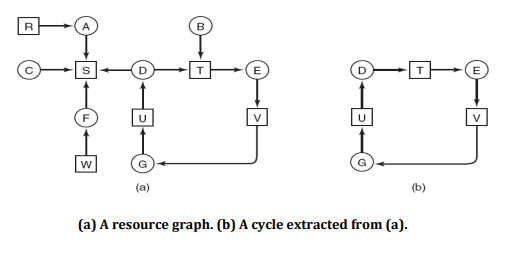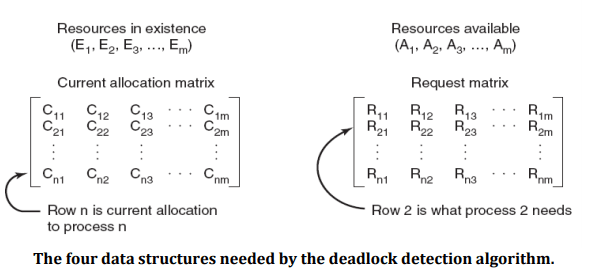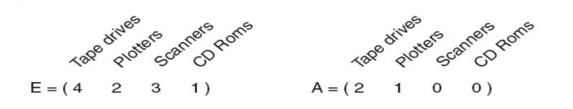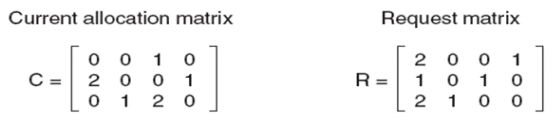Here in this section of Operating System Long Questions and Answers,We have listed out some of the important Long Questions with Answers on Deadlock detection and recovery which will help students to answer it correctly in their University Written Exam.

Lists of Long Descriptive type Questions that may be asked in Written Exams.

• (1) Explain deadlock detection and recovery

Question-1 Explain deadlock detection and recovery

Deadlock detection with single resource of each type.

Algorithm for detecting deadlock for single resource

1. For each node, N in the graph, perform the following five steps with N as the starting node.
2. Initialize L to the empty list, designate all arcs as unmarked.
3. Add current node to end of L, check to see if node now appears in L two times. If it does, graph contains a cycle (listed in L), algorithm terminates.
4. From given node, see if any unmarked outgoing arcs. If so, go to step 5; if not, go to step 6.
5. Pick an unmarked outgoing arc at random and mark it. Then follow it to the new current node and go to step 3.
6. If this is initial node, graph does not contain any cycles, algorithm terminates. Otherwise, dead end. Remove it, go back to previous node, make that one current node, go to step 3.For example as shown in figure above (a),

• We are starting from node D.
• Empty list L = ()
• Add current node so Empty list = (D).
• From this node there is one outgoing arc to T so add T to empty list.
• So Empty list become L = (D, T).
• Continue this step….so we get empty list as below
• L = (D, T, E)………… L = (D, T, E, V, G, U, D)
• In the above step in empty list the node D appears twice, so deadlock.

• When multiple copies of some of the resources exist, a matrix-based algorithm can be used for detecting deadlock among n processes.
• Let the number of resource classes be m, with E1 resources of class 1, E2 resources of class 2, and generally, Ei resources of class i (1 < i < m).
• E is the existing resource vector. It gives the total number of instances of each resource in existence.
• Let A be the available resource vector, with Ai giving the number of instances of resource i that are currently available (i.e., unassigned).
• There are two matrices used in the algorithm. C is a current allocation matrix, and R, the request matrix.• The i-th row of C tells how many instances of each resource class Pi currently holds.
• Thus Cij is the number of instances of resource j that are held by process i.
• Similarly, Rij is the number of instances of resource j that Pi wants.
• These four data structures are shown in Fig.above.

The deadlock detection algorithm can now be given, as follows.

1. Look for an unmarked process, Pi, for which the i-th row of R is less than or equal to A.
2. If such a process is found, add the i-th row of C to A, mark the process, and go back to step 1.
3. If no such process exists, the algorithm terminates.• E is the total no of each resource, C is the number of resources held by each process, A is the number of resources that are available (free), and R is the number of resources still needed by each process to proceed.
• At this moment, we run the algorithm:
• P3 can be satisfied, so P3 completes, returns resources A = (2 2 2 0)
• P2 can be satisfied, so P2 completes, returns resources A = (4 2 2 1)
• P1 can be satisfied, so P1 completes.
• Now all processes are marked, so no deadlock.

Recovery through preemption

• In some cases it may be possible to temporarily take a resource away from its current owner and give it to another process.
• The ability to take a resource away from a process, have another process use it,and then give it back without the process noticing it is highly dependent on the nature of the resource.
• Recovering this way is frequently difficult or impossible.
• Choosing the process to suspend depends largely on which ones have resources that can easily be taken back.

Recovery through rollback

• Create a checkpoint.
• Checkpoint a process periodically.
• Checkpointing a process means that its state is written to a file so that it can be restarted later.
• The checkpoint contains not only the memory image, but also the resource state, that is, which resources are currently assigned to the process.
• When a deadlock is detected, it is easy to see which resources are needed.
• To do the recovery, a process that owns a needed resource is rolled back to a point in time before it acquired some other resource by starting one of its earlier checkpoints.
• In effect, the process is reset to an earlier moment when it did not have the resource, which is now assigned to one of the deadlocked processes.
• If the restarted process tries to acquire the resource again, it will have to wait until it becomes available.

Recovery through killing processes

• The crudest, but simplest way to break a deadlock is to kill one or more processes.
• One possibility is to kill a process in the cycle. With a little luck, the other processes will be able to continue.
• If this does not help, it can be repeated until the cycle is broken.
• Alternatively, a process not in the cycle can be chosen as the victim in order to release its resources.
• In this approach, the process to be killed is carefully chosen because it is holding resources that some process in the cycle needs.

You may be interested in:
Operating System Short Descriptive Questions and Answers

Operating System Multiple Choice Questions Answers (MCQs)

Operating System Online Tests

Windows Operating System Online Tests

Linux Operating System Online Tests